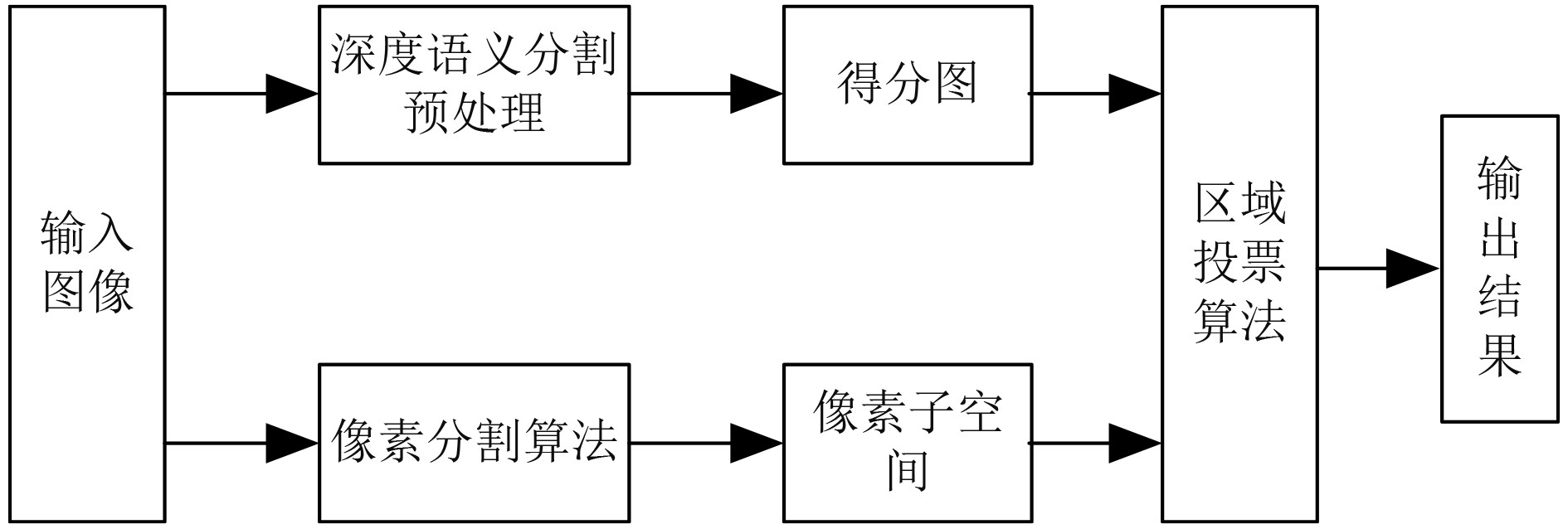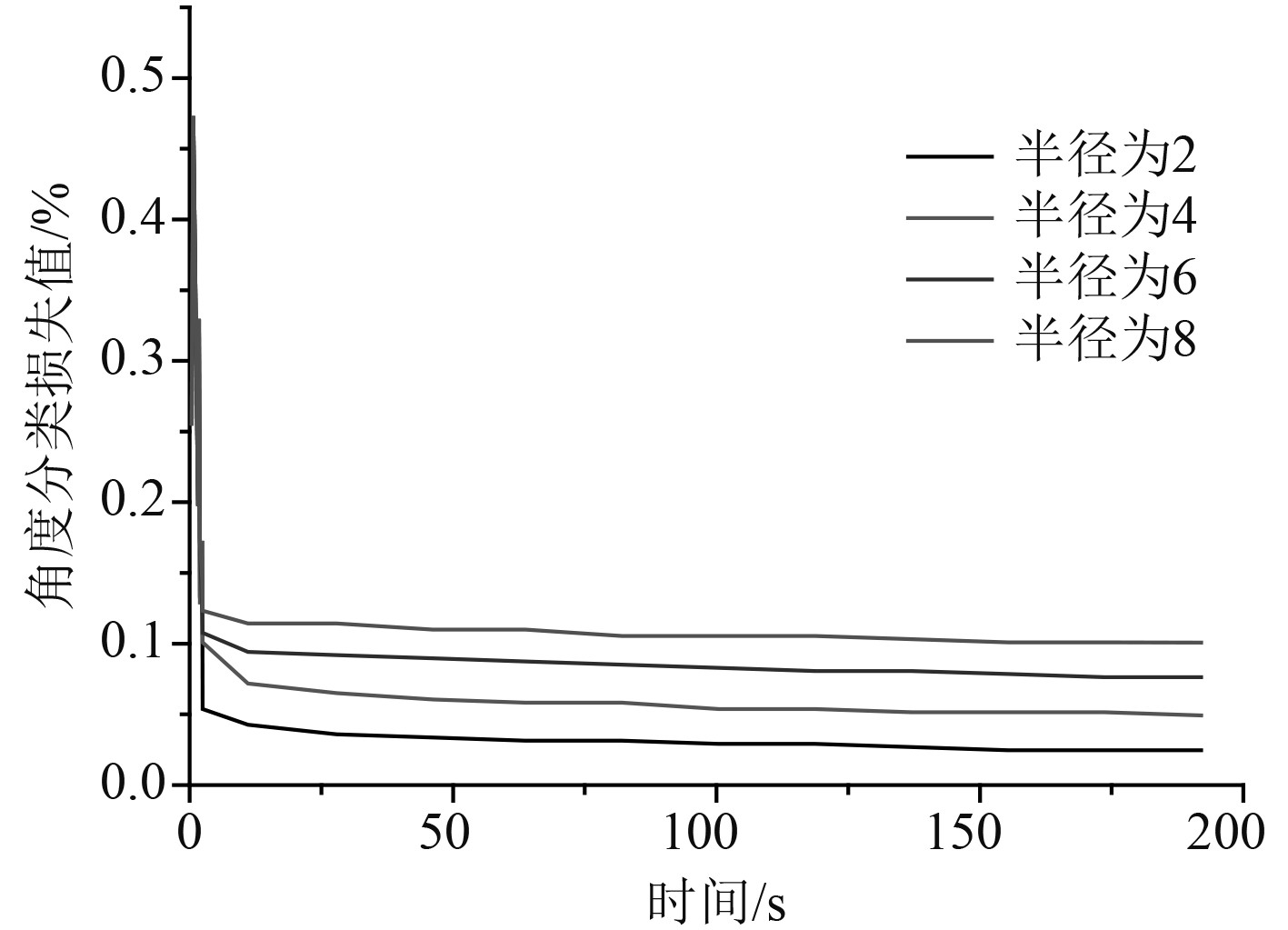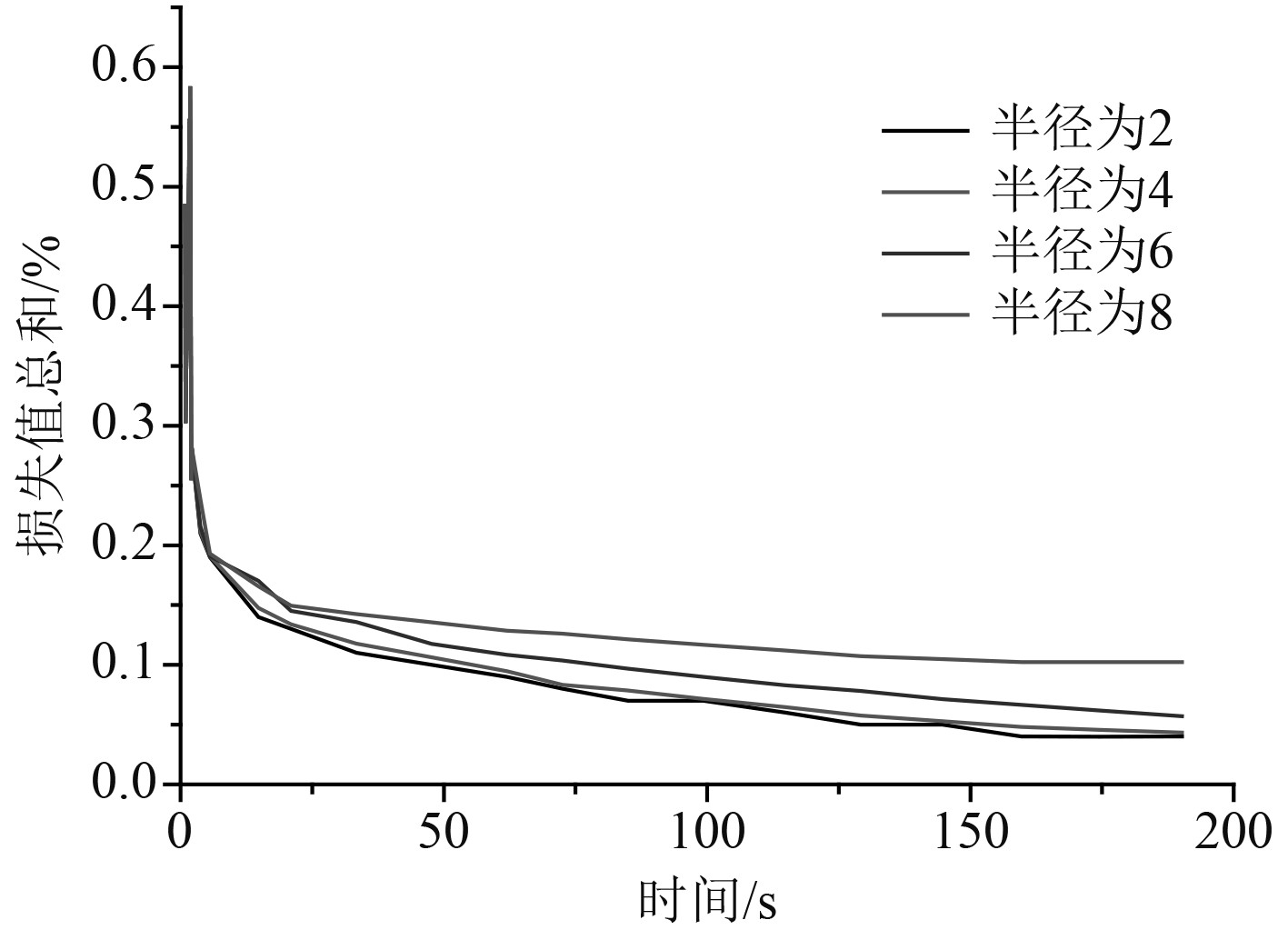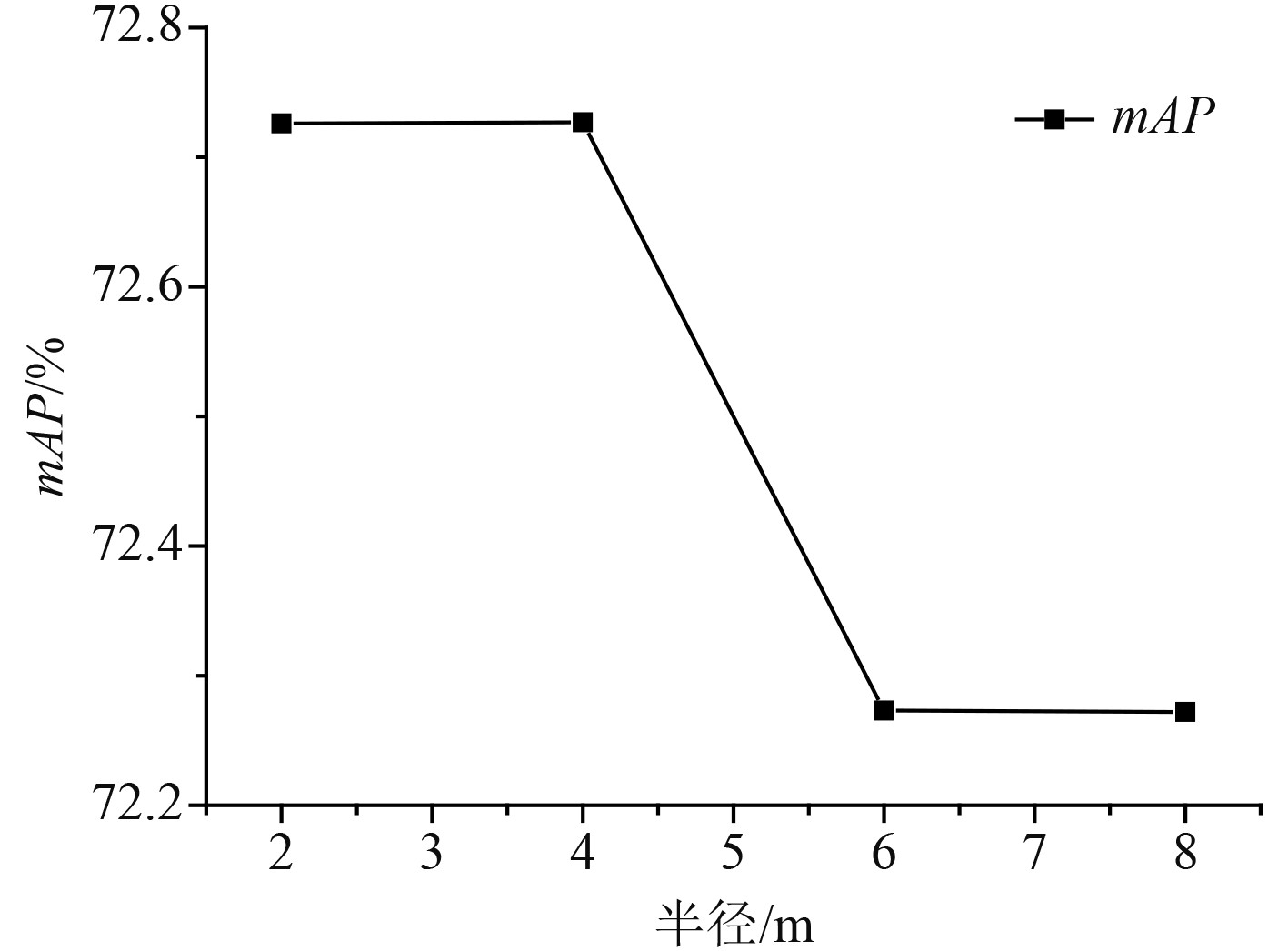﻿ 语义分割技术在船舶卫星图像识别中的应用
 舰船科学技术2022, Vol. 44Issue (14): 155-158    DOI: 10.3404/j.issn.1672-7649.2022.14.033PDF

1. 河南大学 濮阳工学院，河南 濮阳 457000;
2. 濮阳职业技术学院，河南 濮阳 457000

Application of semantic segmentation technology in ship satellite image recognition
ZHENG Shuai1,2
1. Puyang Institute of Technology, Henan University, Puyang 457000, China;
2. Puyang Vocational and Technical College, Puyang 457000, China
Abstract: On the basis of analyzing the limitations of traditional image segmentation algorithms, a new ship satellite image recognition method based on semantic segmentation technology is studied. The evaluation criteria of image semantic segmentation technology are proposed, including pixel precision, average pixel precision and average pixel precision. The angle loss of different Gaussian function radii is analyzed, and the loss values of single hot code and Gaussian function are compared.
Key words: semantic segmentation technology     warship     satellite image recognition
0 引　言

1 图像语义分割技术 1.1 图像分割方法

 $g\left( {i,j} \right) = \left\{ {\begin{array}{*{20}{c}} {1,f\left( {i,j} \right) \geqslant T}，\\ {0,f\left( {i,j} \right) < T} 。\end{array}} \right.$ (1.1)

1.2 基于深度学习的图像语义分割法图 1 基于区域投票算法的图像语义分割技术 Fig. 1 Image semantic segmentation based on region voting algorithm

 $P\left( x \right) = \frac{1}{N}\sum\limits_{i = 0}^N {k\left( {x - {x_i}} \right),x \subseteq {R^d}} 。$ (1.2)

 ${y_i}\left( {t + 1} \right) = \frac{{\displaystyle\sum\limits_{j = 1}^N {\psi \left( {\left\| {{y_i}\left( t \right) - {x_j}} \right.\left\| {_2^2} \right.} \right){x_j}} }}{{\displaystyle\sum\limits_{j = 1}^N {\psi \left( {\left\| {{y_i}\left( t \right) - {x_j}} \right.\left\| {_2^2} \right.} \right)} }}。$ (1.3)

 ${p_i} = \frac{1}{N}\sum\limits_{j = 1}^N {\phi \left( {{D_{ij}}} \right)} 。$ (1.4)

 $\Pr ed\left( S \right) = k,if\# label\left( k \right) \gt \# label\left( i \right),\forall i \in \left[ {0,{N_c} - 1} \right] 。$ (1.5)

 $H\left( S \right) = - \sum\limits_{i = 0}^{{N_c} - 1} {p\left( {label\left( i \right)} \right)\log \left( {p\left( {label\left( i \right)} \right)} \right)} 。$ (1.6)

 $p\left( {label\left( i \right)} \right) = \frac{{\# label\left( i \right)}}{M}。$ (1.7)

 $F\left( S \right) = \left\{ {\begin{array}{*{20}{c}} {1,}&{\begin{array}{*{20}{c}} {\rm if}&{H\left( S \right) < {{T}}}，\end{array}} \\ {0,}&{\rm otherwise} 。\end{array}} \right.$ (1.8)

1.3 图像语义分割评价标准

 $PA = \frac{{\displaystyle\sum\limits_{i = 0}^k {{P_{ii}}} }}{{\displaystyle\sum\limits_{i = 0}^k {\displaystyle\sum\limits_{j = 0}^k {{P_{ij}}} } }}\text{，}$ (1.9)
 $MPA = \frac{1}{{k + 1}}\sum\limits_{i = 0}^k {\frac{{{P_{ii}}}}{{\displaystyle\sum\limits_{j = 0}^k {{P_{ij}}} }}} \text{，}$ (1.10)
 $MIoU = \frac{1}{{k + 1}}\displaystyle\sum\limits_{i = 0}^k {\frac{{{P_{ii}}}}{{\displaystyle\sum\limits_{j = 0}^k {{P_{ij}}} + \displaystyle\sum\limits_{j = 0}^k {{P_{ji}}} - {P_{ii}}}}} 。$ (1.11)

2 舰船卫星图像识别技术 2.1 目标舰船数据集构建及实验指标

 $P = \frac{{TP}}{{TP + FP}}。$ (2.1)

 $R = \frac{{TP}}{{TP + FN}}。$ (2.2)

 $AP = \int_0^1 {P\left( R \right)} {\rm{d}}R 。$ (2.3)

 $mAP = \frac{{\sum {AP} }}{N}。$ (2.4)
2.2 仿真结果及分析图 2 不同高斯函数半径下角度分类损失值 Fig. 2 Loss value of angle classification under different Gaussian function radii图 3 不同高斯函数半径下损失值总和 Fig. 3 Sum of loss values under different Gaussian function radii图 4 不同的高斯函数窗口半径下的mAP Fig. 4 mAP under different window radius of Gaussian function
3 结　语

  刘星璇, 饶世钧, 洪俊. 中低分辨率遥感影像舰船目标识别方法[J]. 舰船电子工程, 2018, 38(16): 21-25. DOI:10.3969/j.issn.1672-9730.2018.06.006  王彦情, 马雷, 田原. 光学遥感图像舰船目标检测与识别综述[J]. 自动化学报, 2011, 37(9): 1029-1039.  刘勇, 姚力波, 吴昱舟, 等. 静轨光学卫星与自动识别系统的目标点迹关联与误差校正[J]. 电子与信息学报, 2018, 40(7): 1546-1552.  张晓, 王莉莉. 基于多分类器融合的卫星图像舰船目标识别[J]. 通信技术, 2019, 52(9): 2143-2148. DOI:10.3969/j.issn.1002-0802.2019.09.013  孙明珠, 贾丽, 吴翎. 高分辨率SAR卫星图像舰船目标识别可用性分析[J]. 佳木斯大学学报, 2021, 39(1): 131-133.  李家起, 江政杰, 姚力波, 等. 一种基于深度学习的舰船目标融合识别算法[J]. 舰船电子工程, 2020, 40(9): 31–35.  邬厚民. 基于人工智能技术的舰船图像语义分割算法[J]. 舰船科学技术, 2020, 42(16): 58-60.